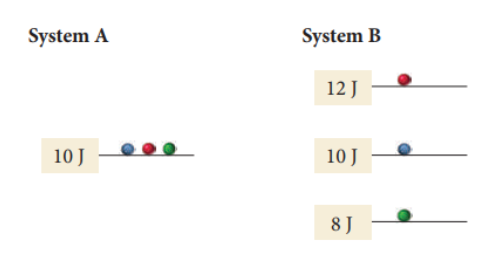# Problem: Two systems, each composed of three particles represented by circles, have 30 J of total energy. How many energetically equivalent ways can you distribute the particles in each system? Which system has greater entropy?

###### FREE Expert Solution

For System A, We only have 1 energy level so there's only 1` way to distribute the particles.

95% (62 ratings)###### Problem Details

Two systems, each composed of three particles represented by circles, have 30 J of total energy. How many energetically equivalent ways can you distribute the particles in each system? Which system has greater entropy?